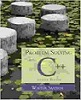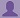SHARE

To start with, Sr2Jr’s first step is to reduce the expenses related to education. To achieve this goal Sr2Jr organized the textbook’s question and answers. Sr2Jr is community based and need your support to fill the question and answers. The question and answers posted will be available free of cost to all.# Problem Solving With C++

Authors: Walter Savitch ,julia Lobur Chapter: More Flow Of Control
ISBN: 9780321531346 Exercise: Self-test Exercises
Edition: 7 Question: 11
 Previous Next

###Question

What output will be produced by the following code, when embedded in a complete program?

int x = SOME_CONSTANT;

cout << "Start\n";

if (x < 100)

cout << "First Output.\n";

else if (x > 100)

cout << "Second Output.\n";

else

cout << x << endl;

cout << "End\n";

SOME_CONSTANT is a constant of type int. Assume that neither

"First Output

###Answer

The output of the given code:

Start

100

End

### Discussions

Post the discussion to improve the above solution.